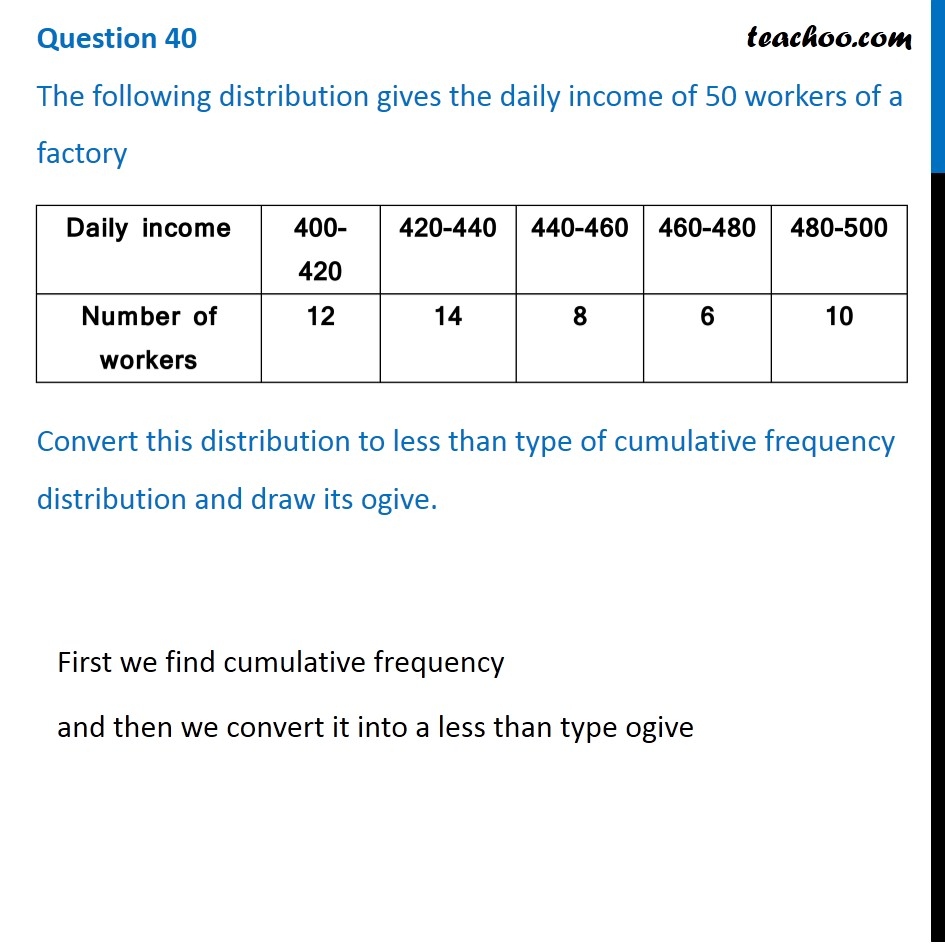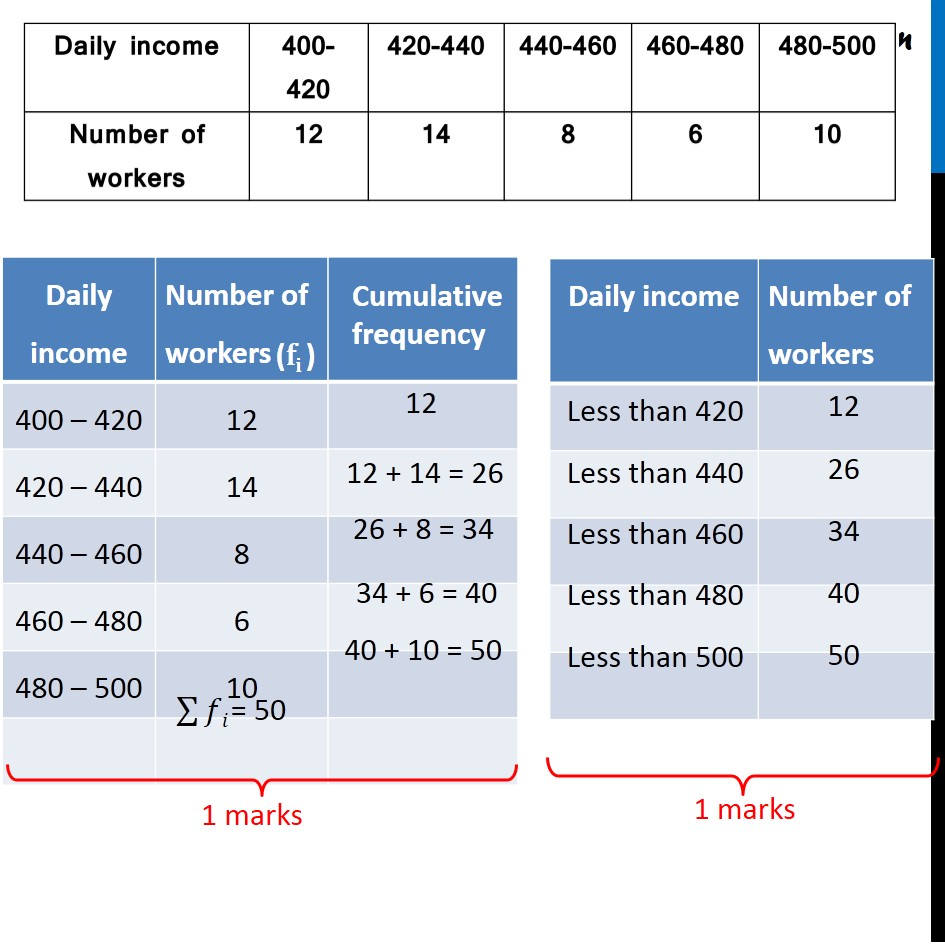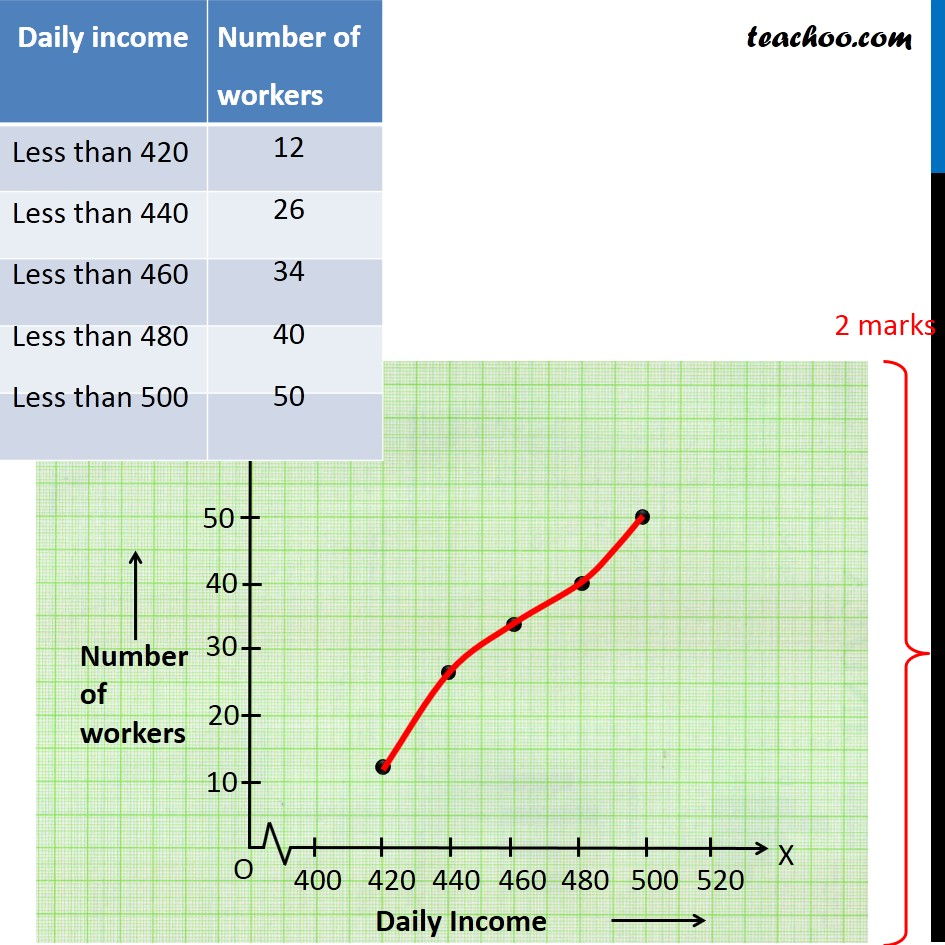## The following distribution gives the daily income of 50 workers of a factory

 Daily Income 400 - 420 420-440 440-460 460-480 480-500 Number of Workers 12 14 8 6 10

## distribution and draw its ogive.Note : This is similar to Ex 14.4, 1 of NCERT – Chapter 14 Class 10

1. Class 10
2. Solutions of Sample Papers for Class 10 Boards
3. CBSE Class 10 Sample Paper for 2020 Boards - Maths Basic

Transcript

Question 40 The following distribution gives the daily income of 50 workers of a factory Convert this distribution to less than type of cumulative frequency distribution and draw its ogive. First we find cumulative frequency and then we convert it into a less than type ogive Daily income Number of workers 400 – 420 12 420 – 440 14 440 – 460 8 460 – 480 6 480 – 500 10 Cumulative frequency 12 12 + 14 = 26 26 + 8 = 34 34 + 6 = 40 40 + 10 = 50 Daily income Less than 420 - 12 Less than 440 - 26 Less than 460 - 34 Less than 480 - 40 Less than 500 - 50

CBSE Class 10 Sample Paper for 2020 Boards - Maths Basic

Class 10
Solutions of Sample Papers for Class 10 Boards